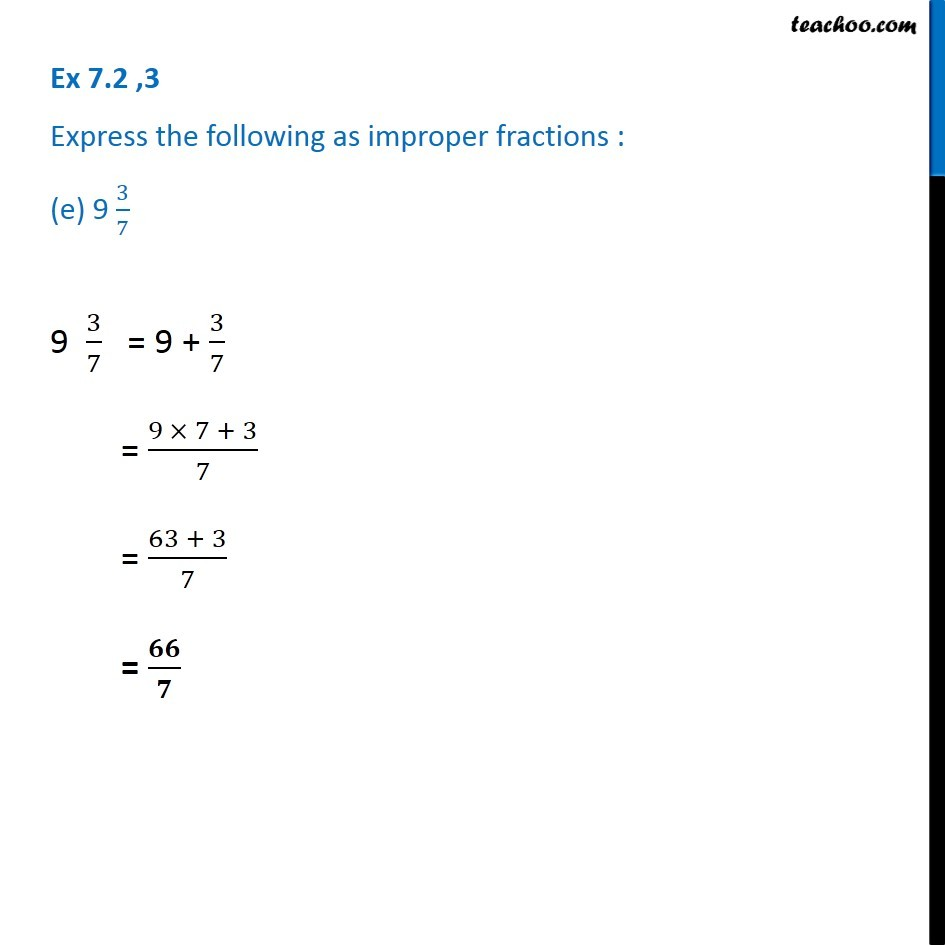1. Chapter 7 Class 6 Fractions
2. Serial order wise
3. Ex 7.2

Transcript

Ex 7.2 ,3 Express the following as improper fractions : (e) 9 3/7 9 3/7 = 9 + 3/7 = (9 × 7 + 3)/7 = (63 + 3)/7 = 𝟔𝟔/𝟕